# ML Aggarwal Solutions for Class 7 Maths Chapter 4 Exponents and Powers

ML Aggarwal Solutions for Class 7 Maths Chapter 4 Exponents and Powers are provided here. The main aim is to help students understand and crack these problems. We, at BYJU’S, have prepared these solutions wherein problems are solved step by step with detailed explanations. Students who wish to score good marks in Maths diligently practise these ML Aggarwal Solutions. Download pdf of Class 7 Chapter 4 in their respective links.

Chapter 4 – Exponents are the product of rational numbers multiplied several times by themselves. This chapter includes laws of exponents, expressing large numbers in standard form, numbers in expanded form, square root. Exponents and Powers solutions are available for download in pdf format and provide solutions to all the questions provided in ML Aggarwal Solutions for Class 7 Maths Chapter 4 Exponents and Powers.

## Download the PDF of ML Aggarwal Solutions for Class 7 Maths Chapter 4 Exponents and Powers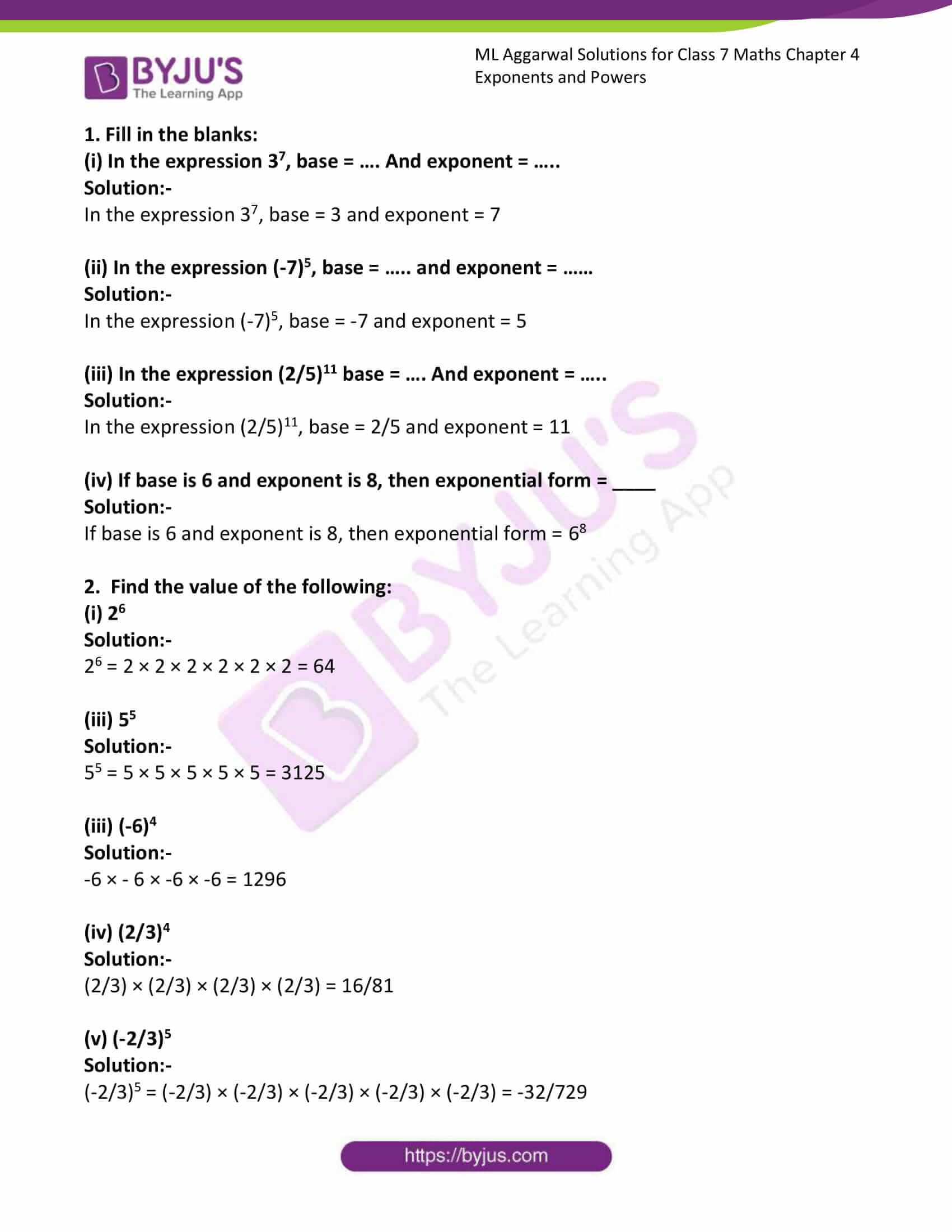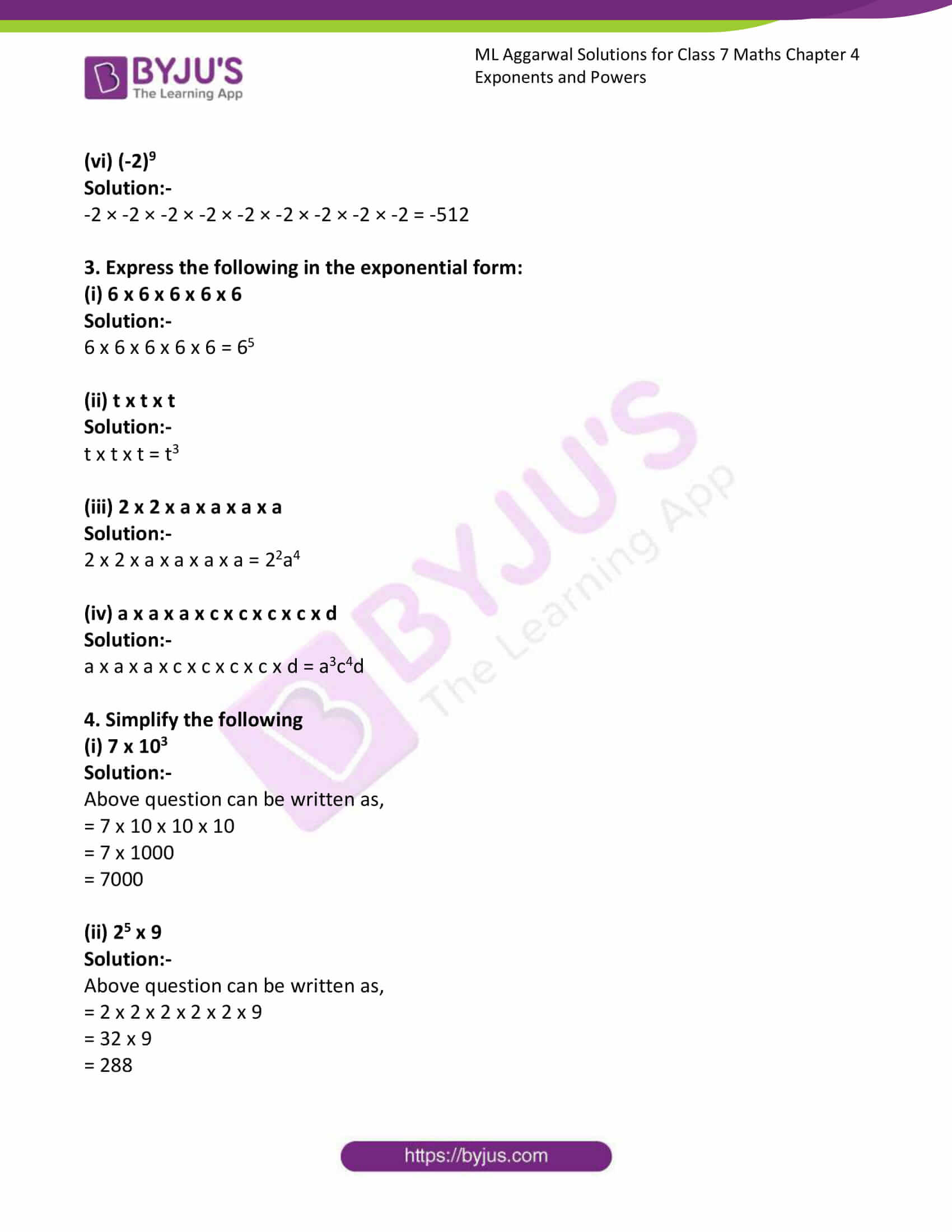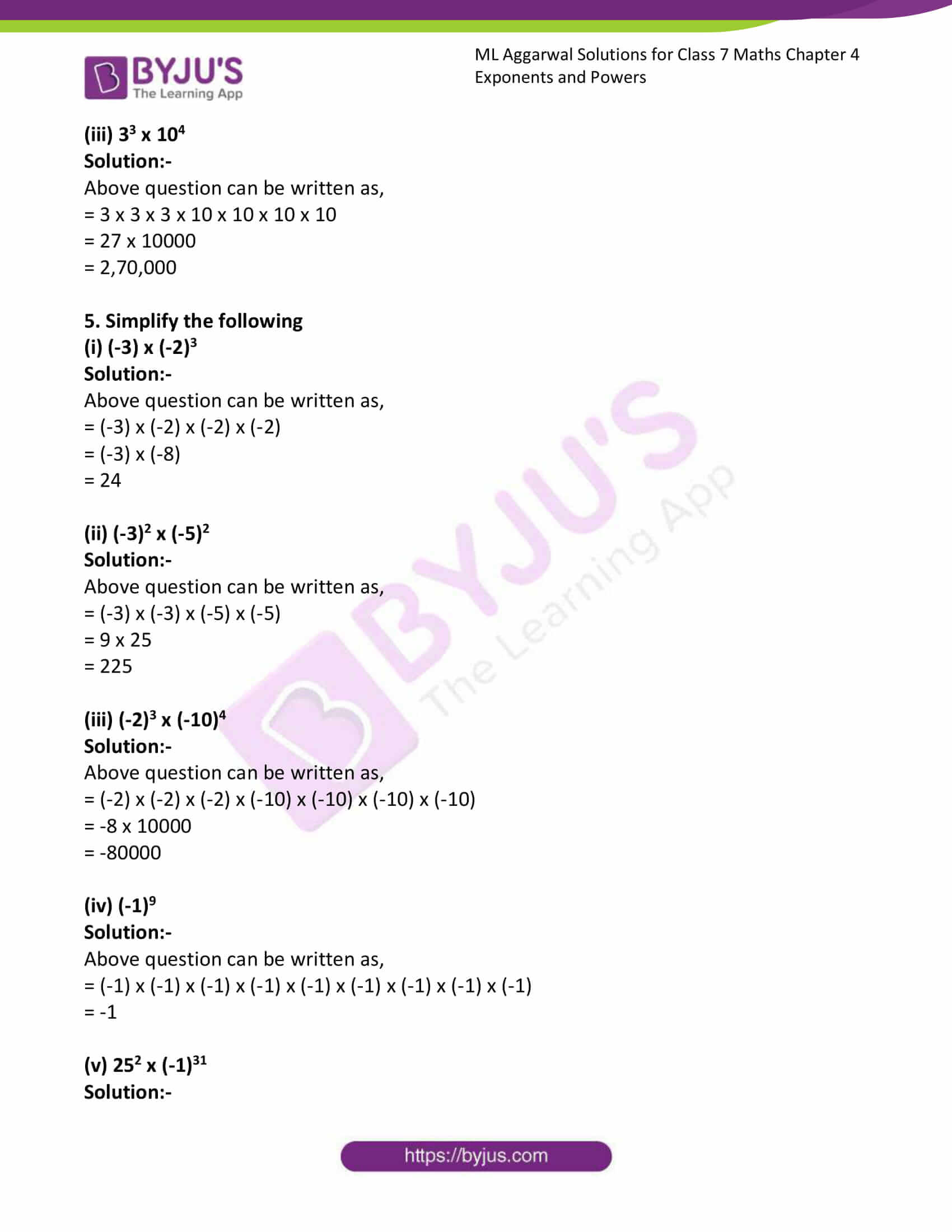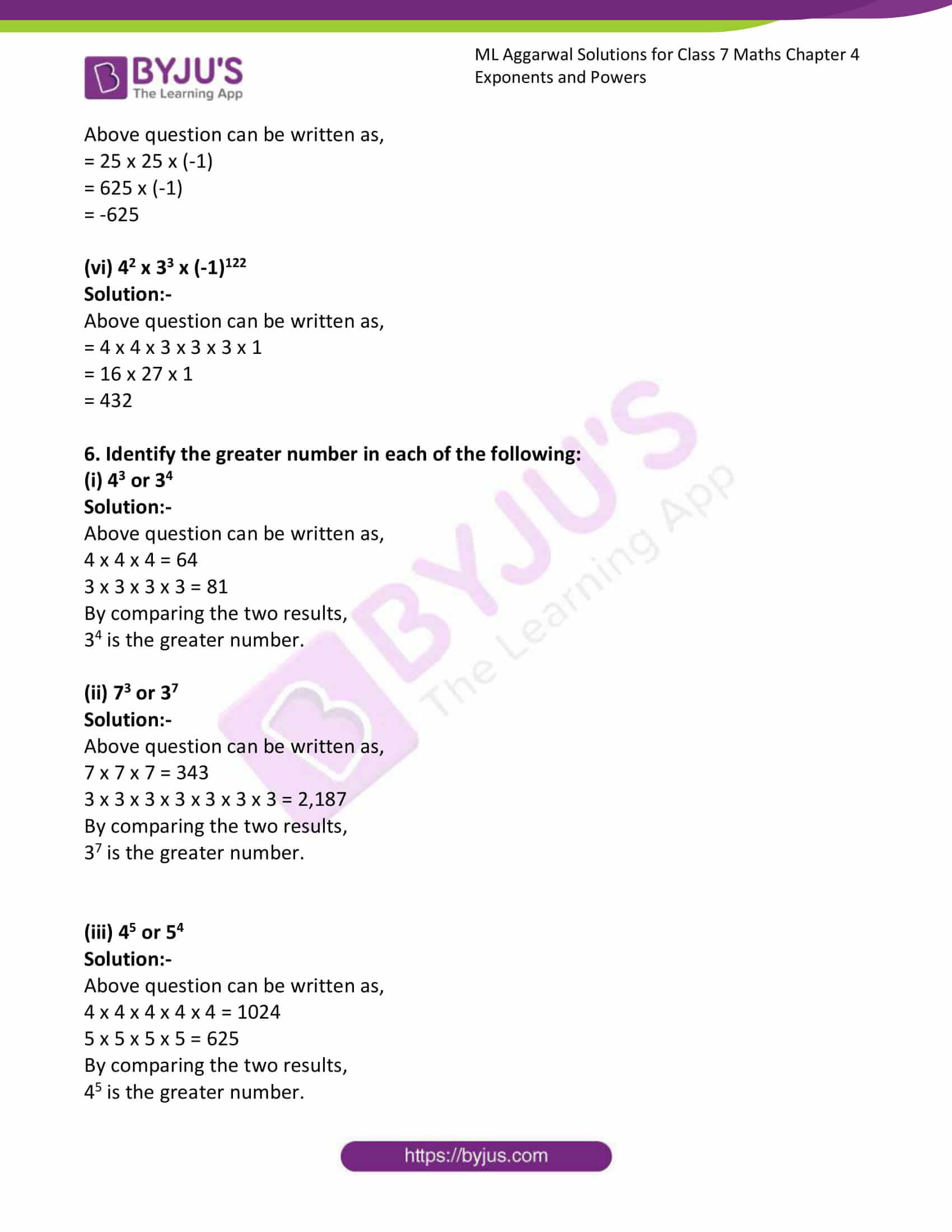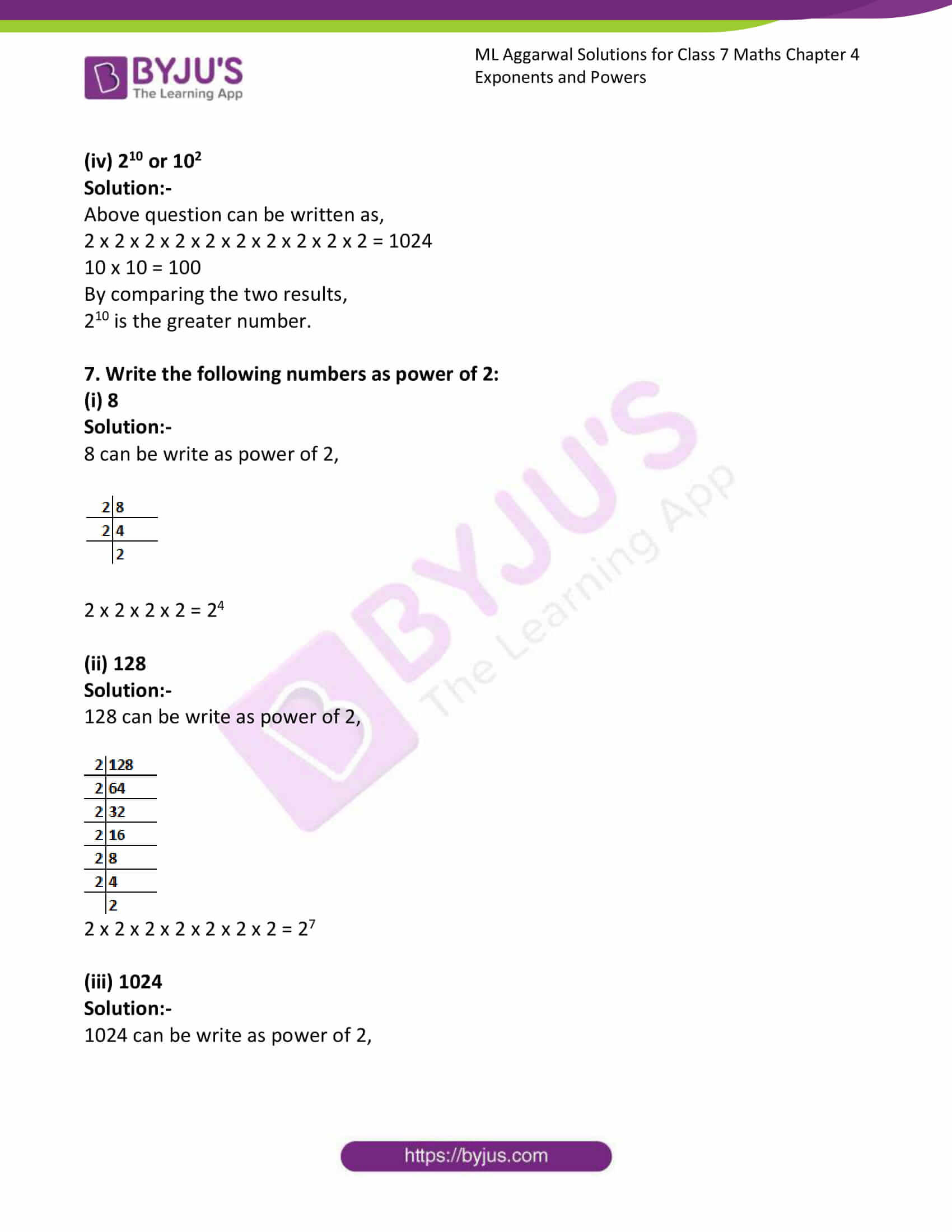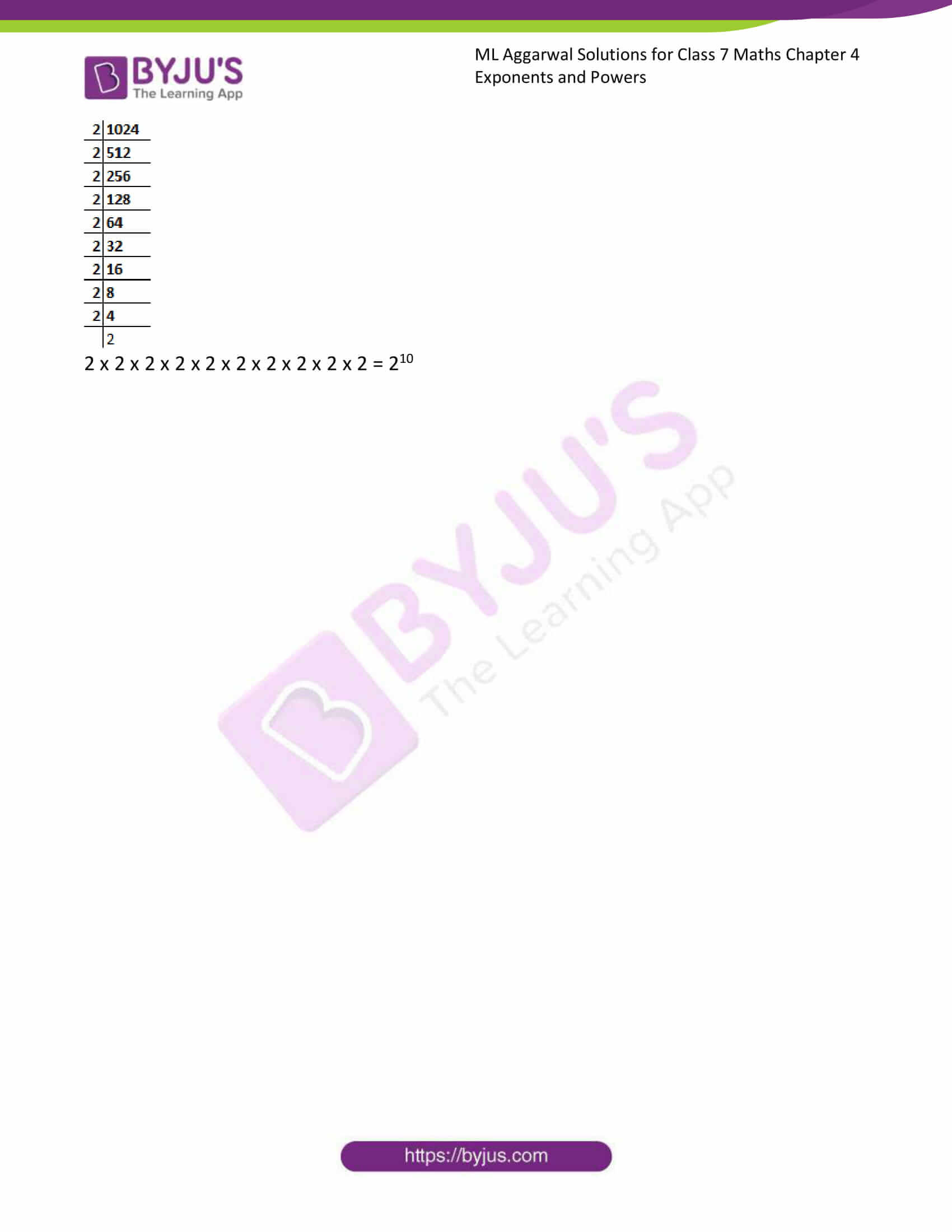### Access answers to ML Aggarwal Solutions for Class 7 Maths Chapter 4 Exponents and Powers

1. Fill in the blanks:

(i) In the expression 37, base = …. And exponent = …..

Solution:-

In the expression 37, base = 3 and exponent = 7

(ii) In the expression (-7)5, base = ….. and exponent = ……

Solution:-

In the expression (-7)5, base = -7 and exponent = 5

(iii) In the expression (2/5)11 base = …. And exponent = …..

Solution:-

In the expression (2/5)11, base = 2/5 and exponent = 11

(iv) If base is 6 and exponent is 8, then exponential form = ____

Solution:-

If base is 6 and exponent is 8, then exponential form = 68

2. Find the value of the following:

(i) 26

Solution:-

26 = 2 × 2 × 2 × 2 × 2 × 2 = 64

(iii) 55

Solution:-

55 = 5 × 5 × 5 × 5 × 5 = 3125

(iii) (-6)4

Solution:-

-6 × – 6 × -6 × -6 = 1296

(iv) (2/3)4

Solution:-

(2/3) × (2/3) × (2/3) × (2/3) = 16/81

(v) (-2/3)5

Solution:-

(-2/3)5 = (-2/3) × (-2/3) × (-2/3) × (-2/3) × (-2/3) = -32/729

(vi) (-2)9

Solution:-

-2 × -2 × -2 × -2 × -2 × -2 × -2 × -2 × -2 = -512

3. Express the following in the exponential form:

(i) 6 x 6 x 6 x 6 x 6

Solution:-

6 x 6 x 6 x 6 x 6 = 65

(ii) t x t x t

Solution:-

t x t x t = t3

(iii) 2 x 2 x a x a x a x a

Solution:-

2 x 2 x a x a x a x a = 22a4

(iv) a x a x a x c x c x c x c x d

Solution:-

a x a x a x c x c x c x c x d = a3c4d

4. Simplify the following

(i) 7 x 103

Solution:-

Above question can be written as,

= 7 x 10 x 10 x 10

= 7 x 1000

= 7000

(ii) 25 x 9

Solution:-

Above question can be written as,

= 2 x 2 x 2 x 2 x 2 x 9

= 32 x 9

= 288

(iii) 33 x 104

Solution:-

Above question can be written as,

= 3 x 3 x 3 x 10 x 10 x 10 x 10

= 27 x 10000

= 2,70,000

5. Simplify the following

(i) (-3) x (-2)3

Solution:-

Above question can be written as,

= (-3) x (-2) x (-2) x (-2)

= (-3) x (-8)

= 24

(ii) (-3)2 x (-5)2

Solution:-

Above question can be written as,

= (-3) x (-3) x (-5) x (-5)

= 9 x 25

= 225

(iii) (-2)3 x (-10)4

Solution:-

Above question can be written as,

= (-2) x (-2) x (-2) x (-10) x (-10) x (-10) x (-10)

= -8 x 10000

= -80000

(iv) (-1)9

Solution:-

Above question can be written as,

= (-1) x (-1) x (-1) x (-1) x (-1) x (-1) x (-1) x (-1) x (-1)

= -1

(v) 252 x (-1)31

Solution:-

Above question can be written as,

= 25 x 25 x (-1)

= 625 x (-1)

= -625

(vi) 42 x 33 x (-1)122

Solution:-

Above question can be written as,

= 4 x 4 x 3 x 3 x 3 x 1

= 16 x 27 x 1

= 432

6. Identify the greater number in each of the following:

(i) 43 or 34

Solution:-

Above question can be written as,

4 x 4 x 4 = 64

3 x 3 x 3 x 3 = 81

By comparing the two results,

34 is the greater number.

(ii) 73 or 37

Solution:-

Above question can be written as,

7 x 7 x 7 = 343

3 x 3 x 3 x 3 x 3 x 3 x 3 = 2,187

By comparing the two results,

37 is the greater number.

(iii) 45 or 54

Solution:-

Above question can be written as,

4 x 4 x 4 x 4 x 4 = 1024

5 x 5 x 5 x 5 = 625

By comparing the two results,

45 is the greater number.

(iv) 210 or 102

Solution:-

Above question can be written as,

2 x 2 x 2 x 2 x 2 x 2 x 2 x 2 x 2 x 2 = 1024

10 x 10 = 100

By comparing the two results,

210 is the greater number.

7. Write the following numbers as power of 2:

(i) 8

Solution:-

8 can be write as power of 2,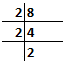2 x 2 x 2 x 2 = 24

(ii) 128

Solution:-

128 can be write as power of 2,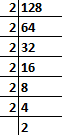2 x 2 x 2 x 2 x 2 x 2 x 2 = 27

(iii) 1024

Solution:-

1024 can be write as power of 2,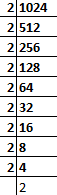2 x 2 x 2 x 2 x 2 x 2 x 2 x 2 x 2 x 2 = 210